# We Helped With This Statistics with R Programming Assignment: Have A Similar One?

SOLVEDCategory Programming R | R Studio Undergraduate Solved Statistics Project Helper

## Assignment Description

GEOG 5670 Spatial Analysis

Homework #5 Hypothesis Tests

The first two problems demonstrate how to do hypothesis tests in R. In this exercise we’ll be using the pastecs, psych, and lsr packages. In RStudio install these (if you haven’t already done so) and check the box next to each to load them.

1.       A proposed fertilizer is being evaluated for the amount by which it increases corn production. It was decided to use a small sample of 12 farms to determine if the fertilizer results in a noticeable increase in corn yields of more than 5 bushels/acre. Based upon similar experiments in the past, the population of yield changes was believed to be normally distributed. The resulting yield changes are:

15.3, 12.9, -3.2, 16.4, 4.3, 14.6, 15.0, -2.1, 15.5, 7.2, 9.1, 15.2

Enter these data into the variable YieldChg (to indicate yield change) using the combine function c(). Use the >describe() function to get the mean and standard deviation of this sample.

a.       Is this a one or two tailed test?

b.       What is the value for mu (m)?

c.       Given the number of samples and the supplied knowledge of the population statistics, what type of test statistic and distribution will you use?

d.       State the null and alternate hypothesis Use the:

t.test(x, y = NULL, alternative = c("two.sided", "less", "greater"),

mu = 0, paired = FALSE, var.equal = FALSE, conf.level = 0.95, ...)

function to run a one-sample t test on x = YieldChg (be sure to specify the hypothesized difference of 5 bushels/acre as mu = 5.0 and the correct alternative = (either “two.sided”, “less”, or “greater”).

e.       What is the value of the test statistic for the yield change of the 12 farms in the study?

f.        What is the p-value for the sample mean?

g.       What is the 95% confidence interval for the difference in yield changes between your sample and the 5 bushel/acre difference? Since this interval does not include 5, what does this indicate about the significance of the difference between your group and the 5 bushel/acre yield change you specified in the t.test?

h.       What do you conclude with regard to the null and alternate hypotheses?

2.       A local auto dealer wants to know whether single male buyers purchase the same amounts of options as do single females when ordering a new car. A sample of eight males and ten females was obtained. The data consist of the amounts of the ordered extras in hundreds

 Males Females 23.00 16.42 23.86 14.20 19.20 21.30 15.78 18.46 30.65 11.70 23.12 12.10 17.90 16.50 30.25 9.20 21.05 18.05

This data is on the course elearning page as autos.csv. This file consists of two columns, Gender and Options and is thus in “long” format. Use the read.csv() function in R to read in the data to a dataframe called carOptions

a.       State the null and alternate hypothesis

b.       What sampling statistic and distribution will you be using to evaluate this hypothesis? (assume the population variances are equal)

c.       Is this a one or two-tailed test?

Use the t.test() function to run an independent samples t-test to evaluate your hypothesis. Since this is long form data in which there is one column for the options purchased, you will need to specify a formula to separate the males and females based on the Gender factor. The input argument to the t-test() function will be a formula Options ~ Gender with the data argument set to the carOptions dataframe. Since “two.sided” is the default, it is not necessary to specify this argument. For now, set the var.equal option to TRUE.

d.       What is the value of the test statistic when equal variances are assumed? What is the p-

value for the equal variance assumption?

e.       What is the 95% confidence interval (equal variances assumed) for the difference in the cost of options ordered by males and females?  Since this interval does not include zero, what does this indicate about the significance of the difference between males and females?

f.        What is the mean difference between male expenditures on options and female expenditures? Who spends more?

g.       What is your decision regarding the hypothesis?

Run the t-test again, this time with the var.equal argument set to FALSE.

h.       What is the value of the test statistic when equal variances are not assumed?

i.        What is the p-value for the unequal variance assumption? What is your decision regarding the hypothesis?

j.        How do the number of degrees of freedom compare between the equal and unequal variance assumptions?

k.       For the unequal variance assumption, is the 95% confidence interval wider or narrower than the equal variance assumption? What does this indicate about the power of the independent samples t-test under the assumptions of equal and unequal variances?

3.       An exercise program claims to reduce weight by more than 20 pounds. A test of this claim was made by selecting a group of eight people and checking their weight before and after the program. Enter the values below into a Before vector and an After vector using c(). You can either use these separate vectors as input to the t.test() function or you could turn them into a dataframe using the data.frame() function and use the \$ notation to designate the input arguments to the t.test() function. Be sure to set the paired argument to TRUE.

 Weight Before Weight After 145 115 160 130 119 100 132 109 175 165 145 125 125 101 132 105

a.       State the null and alternate hypothesis

b.       Is this a one or two tailed test?

c.       What is the value of the test statistic?

d.       What is the p-value for the sample mean?

e.       What is the 95% confidence interval for the mean weight lost?

f.        Does this interval include the hypothesized value (20 lbs), and based on this, is the program's claim of a 20 pound reduction valid?

What do you conclude with regard to the null and alternate hypotheses?

GEOG 5670 Spatial Analysis

### Homework #5

1.

a.       Is this a one or two tailed test?

b.      What is the value for mu (m)?

c.       Given the number of samples and the supplied knowledge of the population statistics, what type of test statistic and distribution will you use?

d.      State the null and alternate hypothesis

e.       What sampling statistic and distribution will you use to evaluate this hypothesis?

f.        What is the value of the test statistic for the yield change of the 12 farms in the study?

g.      What is the p-value for the sample mean?

h.      What is the 95% confidence interval for the difference in yield changes between your sample and the 5 bushel/acre difference?  Since this interval does not include 5, what does this indicate about the significance of the difference between your group and the 5 bushel/acre yield change you specified in the t.test?

2.

a.       State the null and alternate hypothesis

b.      What sampling statistic and distribution will you be using to evaluate this hypothesis? (assume the population variances are equal)

c.       Is this a one or two-tailed test?

d.      What is the value of the test statistic when equal variances are assumed? What is your decision regarding the hypothesis?

e.       What is the p-value for the equal variance assumption?

f.        What is the 95% confidence interval for the difference in the cost of options ordered by males and females?  Since this interval does not include zero, what does this indicate about the significance of the difference between males and females?

g.      What is the mean difference between male expenditures on options and female expenditures?  Who spends more?

h.      For the unequal variance assumption, is the 95% confidence interval wider or narrower than the equal variance assumption?  What does this indicate about the power of the independent samples t-test under the assumptions of equal and unequal variances?

i.        What is the value of the test statistic when equal variances are not assumed?

j.        What is the p-value for the equal variance assumption? What is your decision regarding the hypothesis?

k.      How do the number of degrees of freedom compare between the equal and unequal variance assumptions?

l.        What is the 95% confidence interval for the difference in the cost of options ordered by males and females?  How does the width of this confidence interval compare to the equal variance t-test?

3.

a.       State the null and alternate hypothesis

b.      Is this a one or two tailed test?

c.       What is the value of the test statistic?

d.      What is the p-value for the sample mean?

e.       What is the 95% confidence interval for the mean weight lost?

f.        Does this interval include the hypothesized value (20 lbs), and based on this, is the program's claim of a 20 pound reduction valid?

## Assignment Description

PREVIEW

### 1 AND 2-SAMPLE TESTS

Hypothesis Testing

 Tests concerning                                              and

 p-values

 Statistical significance

 Two sample tests

 Difference of means and proportions

 Confidence intervals for differences

 Equality of variances

 RESEARCH VS. STATISTICAL HYPOTHESES CLASSICAL HYPOTHESIS TESTING  Research hypotheses are substantive, testable scientific claims  A research hypothesis is a knowledgeable statement that is tentatively advanced to account for particular scientific facts.   It is a testable idea or testable question on some phenomenon of interest.  It can be investigated by recording facts (data) on the phenomenon of interest.A statistical hypothesis is a statement concerning one or more data distributions or concerning one or more parameters of a distribution.Usually two statistical hypotheses are formulated. These two statistical hypotheses should be mutually exclusive and mutually exhaustive meaning that:   We want to make an inference about some population parameter qWe hypothesize a value q = q0Collect random sample of size n, xqˆ1, x2, …, xn                ˆCalculate point estimator                                       qEvaluate hypothesis to determine whether         does or does not support contention that        q = q0

 There is no overlap between the two statements (mutually exclusive) so that only one of the statements can be true, and;

 The two statements should cover all conceivable possibilities (mutually exhaustive).

 SIX STEPS IN CLASSICAL HYPOTHESIS TESTING FORMULATING HYPOTHESES

 Formulation of hypothesis

 There are two parts to any hypothesis (H)

 Specification of sample statistic and its sampling distribution  H0 is the null hypothesis, or what we are claiming is the value of q

 Selection of a level of significance                      H is the alternate hypothesis, which we accept if the null hypothesis is not true

 A  FormsA B C H0 : q = q HA : q  q H0 : q  q HA : q  q H0 : q  q HA : q  q

 Construction of a decision rule

 Compute value of the test statistic

 Decision

 A CAVEAT HYPOTHESIS FORMS

 You cannot accept H0, you can only reject it with a possibility of being incorrect (Type I error) of a

 Nothing can be proven with hypothesis tests, we can only disprove some things and we can do that only with some chance of error (a)

 A B C H0 : q = q HA : q  q H0 : q  q HA : q  q H0 : q  q HA : q  q A B C H0 : q = q HA : q  q H0 : q  q HA : q  q H0 : q  q HA : q  q

 Form A is a two-sided or non-directional test

 Forms B and C are directional

 B is a lower tail test

 C is an upper tail test

 ONE VS. TWO-TAILED TESTS SELECTION OF SAMPLE STATISTIC

 H0 and HA must be mutually exclusive and exhaustive

 Hypotheses in the forms of B or C ask different questions than A

 One-tailed test are more powerful (1 - b) than twotailed tests, since we don’t have to divide a by two

 Population Parameter Point Estimator Formula for Point Estimate X 1 nx=xi n i=1 Median 50th Percentile 25% Trimmed Mean Mean of middle 50% of samples 10% Trimmed Mean Mean of middle 80% of samples P P = x/n where x = # of successes in n trials S2 1 ns2 =xi x)2 n 1 i=1

 Use the minimum error estimator (least MSE) of the population parameter under study

PROBABILITIES OF MAKING INCORRECT

DECISIONS

 The level of significance of a classical test of hypothesis is the value chosen for a, the probability of making a Type I error

 Generally a small number like 0.1, 0.05 or 0.01

 Since a is small, we are saying that if we reject H0 we do so with only a small error

 The null hypothesis is something we want to reject, rather than something we want to confirm

 Always report level of significance with result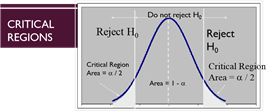p = A1              = 0         A2 - critical values

 The critical region corresponds to those values for which the null hypothesis is rejected

 The less extreme (more central) limits of the critical region are the critical values

### INFERENTIAL ERRORS

 Decision True state  of nature Fail to reject Reject H0 H0 is true No error (1 – a) Type I error (a) H0 is false Type II error (b) No error (1-b)

 Type I error occurs when one rejects a null hypothesis that is actually true

 Probability of committing a Type I error is denoted a

 Type II error occurs when one accepts a null hypothesis that is actually false

 Probability of making a Type II error is denoted b

 SCHEMATIC OF TYPE II ERROR HYPOTHESIS TESTS OF ,           IS KNOWN

 Efficiency of a test at correctly rejecting a false null hypothesis is (1 - b) and is called the power of the test

#### SCHEMATIC OF TYPE I  ERROR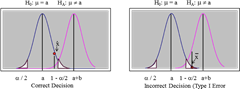Fail to Reject H0                                                                                                         Reject H0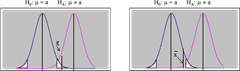a /         a     1 - a/2   a+b                                   a /         a     1 - a/2   a+b

Correct Decision Incorrect Decision (Type II Error) Reject H0                        Fail to Reject H0

 Sample mean statistic 𝑋ത is approximately normally distributed with mean

 and standard deviation

We can evaluate tests of hypotheses concerning using the standard normal statistic

Z=

 Z-tests are rarely used, since you almost never know     and

 No standard z-test function in R, so you have to construct it manually

 Z-TEST IN R Z-TEST ASSUMPTIONS >sample <- c(50, 60, 60, 64, 66, 66, 67, 69, 70, 74, 76, 76, 77, 79, 79, 79, 81, 82, 82, 89) >sample.mean <- mean(sample) >sample.mean  72.3 mu.null <- 68 > sd.true <- 10 > N <- length(sample) > sem.true <- sd.true / sqrt(N) > z.score <- (sample.mean - mu.null) / sem.true> z.score 1.923018 > upper.area <- pnorm(q = z.score, lower.tail = FALSE) > upper.area  0.02723887 > lower.area <- pnorm (q = -z.score, lower.tail = TRUE) > lower.area 0.02723887 > p.value = lower.area + upper.area p.value  Normality Sampling distribution of the mean is normalIndependence No relationship in sample observationsTrue population standard deviation is known Always wrong

 0.05447773

##### STUDENT’S T-TEST

 Hypothesis Tests of ,    is unknown

If                 is unknown, we must use the estimator s and the tdistribution with n-1 degrees of freedom

t =

 This assumes X is normal, or if it is not, we can use a large n (> 30)

 For large n, t-test becomes a z-test, because t-distribution with large n approximates a standard normal distribution

##### HYPOTHESIS TESTING AND CONFIDENCE INTERVALS

 The significance level of a hypothesis test (a) is the complement of the confidence in the confidence interval (1 - a)

 If the (1 - a) confidence interval does not contain the hypothesized value q0 we can reject the hypothesis that H0: q = q0 at the alevel of significance  If the (1 - a) confidence interval includes q0 we cannot reject H0: q = qat the alevel of significance

 Every confidence interval is a two-sided hypothesis test

TWO-SAMPLE TESTS

 Rather than compare a sampling statistic to the population parameter, we sometimes have to compare two samples to see whether they differ

 This is a two-sample test

 We distinguish between the two populations with subscripts:  1and X1 and X2                   n1 and n2

 Sample values get a double subscript xij

 The first subscript, i is the population from which the sample has been drawn

 The second subscript, j is the jth sample

 HYPOTHESES ABOUT AND 1 A B C D H0 : 1 =  HA : 1 H0 : 1   HA : 1 H0 : | 1  HA : | 1D0   D0 H0 : 1    D0 HA : 1    D0

 There are two-sided (Form A) and one-sided (B) tests

 Two-sided version is used when there is no prior information about the direction of the difference

 Forms C and D involve a difference exceeding some specified value D0

 Form C is two-sided, form D is one-sided

 If D0 = 0, we have Forms A and B

INDEPENDENT SAMPLE TESTS

 To decide whether the population means differ we use the difference between the sample means x1 - x2

 We need to know the sampling distribution of the random variable X1 - X2 so we can assign a probability to the results

 We almost never know the population variances, but we can determine whether they are equal

T STATISTIC FOR TWO-SAMPLES

t =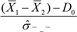X1 X2

 Numerator compares differences in sample means to the hypothesized difference D0

 The denominator is an estimate of the standard deviation of the difference in sample means

##### TEST FOR EQUALITY OF VARIANCES

 If population variances are different, we should not use the pooled variance estimate  Assume X1 and X2 are normally distributed with variances 21 and 22

Given independent random samples of size n1 and n2 then the statistic F=S122122

S22

will follow an F distribution with n1 - 1 and n2 - 1 degrees of freedom

##### LEVENE’S RATIO OF VARIANCES HYPOTHESIS

2                                                                 2

H0 :12 =1            HA :12     1

2                                                                2

 The ratio of the sample variances S21 / S22 is distributed like F

 All F values are greater than 1, so we have to divide the larger sample variance by the smaller, so the ratio can’t be less than 1

 If we reject H0, we use the separate variance formula

 If we can’t reject H0, we should use both the pooled and separate variance estimates (SPSS gives you both by default)

 This test requires the random variables X1 and X2 to be normally distributed

POPULATION VARIANCES EQUAL—

STUDENT INDEPENDENT SAMPLE T-TEST

 There is a single population variance  = 1 =

 We have two sample variances s21 and s22 each of which estimates

 The pooled variance estimate is:      2s2

sp =2

(n1 1) (n2 1)

 This is a weighted average of the two sample variances  The appropriate estimate for      ˆX  1  X   2

(the standard error of the difference) is:                 ˆ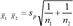SAMPLING DISTRIBUTION OF X1 - X2

EQUAL POPULATION VARIANCES

 Assume X1 and X2 are normal with a difference in

means 1 = D0

 If the variance 2 is the same for both populations, then

the following has the t-distribution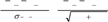(X1                                   X2)                   D0                                       (X1                                     X2)                   D0 t =               =

ˆX1 X2                                 sp 1/n1 1/n2

.

with degrees of freedom

df = n1 + n2 - 2

SAMPLING DISTRIBUTION OF X1 - X2 UNEQUAL

POPULATION VARIANCES (WELCH TEST)

 Assume X1 and X2 are normal with a difference in means 1

= D0 and variances 1

 Then the following has the t-distribution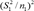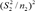.

 An alternate way to estimate df is

 df = min(n1 - 1, n2 - 1)

##### STUDENT’S VERSUS WELCH’S T-TEST

 If you can believe the variance in the two groups is the same, the Student test is more powerful (lower Type II error)

 If the groups do not have the same variance (i.e. no homogeneity of variance), the assumptions of Student’s test are violated, and Welch’s is more appropriate

 You have lower degrees of freedom

 Assuming independent random sampling, the best unbiased point estimator for the difference is X1 - X2

 The confidence intervals will rely on the t-distribution and will have the form

.                   x1     x  2              ta/2 ˆX  1   X                   2

.

with the confidence level 1 - a

 The t-value is multiplied by     ˆX    1 X2    which depends on the assumption of equal variances

 In the lsr package in R, the ciMean() function computes a conf = x confidence interval

 Often in experiments, the two groups are paired on a sample-by-sample basis, possibly as a pre- and posttreatment

 n in this case is the number or pairs

 With matched pairs, the difference between corresponding samples is the random variable of interest

dj = x1j - x2j

 PAIRED OBSERVATIONS SAMPLING DISTRIBUTION OF PAIRED OBSERVATION MEAN

 Assume X1 and X2 are normal with a difference in

 The standard deviation of the differences is   means 1                  = D0

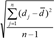Given a random sample of n paired observations, the following has an approximate t-distribution

####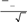D    D0

sd =t =

Sd / n with n - 1 degrees of freedom

 CONFIDENCE INTERVALS FOR 1 - PAIRED OBSERVATIONS

 with the mean difference   n                        Paired observation techniques have much more d j                power than the independent sample tests and cand = j=1                                                                                                  detect smaller significant differences

n

 WIDE AND LONG FORM TABLES IN R RUNNING T-TESTS IN R

 Wide form is the familiar ”case by variable’  layout in which one record or row for every individual, columns represent different attributes for each individual

 Long form is when each row corresponds to a unique measurement             The lsr package has separate oneSampleTTest(), independentSamplesTTest(), and pairedSamplesTTest() functions

reshape(data, varying = NULL, v.names = NULL, timevar =

"time",                                                                                                                                                                                                                                                                                        A more common function from the base R package is t.test()

idvar = "id", ids = 1:NROW(data),

times = seq_along(varying[]),                                   > t.test(x, y = NULL, alternative = c("two.sided", "less", "greater"), drop = NULL, direction, new.row.names = NULL, mu = 0, paired = FALSE, var.equal = FALSE, conf.level = 0.95, ...) sep = ".",

split = if (sep == "") {               t.test() expects data in wide form list(regexp = "[A-Za -z][0-9]", include = TRUE)

} else { list(regexp = sep, include = FALSE, fixed = TRUE)}

)

 EFFECT SIZE EVALUATING ASSUMPTIONS

 Cohen’s d

 d = ((mean 1) – (mean 2)) / std. dev

 Mean 2 is the population mean in one sample tests

 Standard deviation varies, depending on whether you are using pooled standard deviation in a Student’s test, averaged stdev in a Welch’e test, or if you are using only one of the standard deviations in a control group comparison

 cohensD() function in the lsr package

 Also included in oneSampleTTest(), independentSamplesTTest(), pairedSamplesTTest() output

 d-value Rough interpretation ~ 0.2 “small” effect ~ 0.5 “moderate” effect ~0.8 “large” effect

 Interpretation of d is somewhat subjective:

PREVIEW

 Wednesday:  ANOVA lecture

 Read Chapter 10 of the book

 Homework #5 due Wednesday

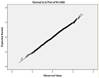Normality:

 QQ Plots

 Histogram shape

 Skewness and Kurtosis statistics

 Shapiro-Wilks or Kolmogorov-Smirnov tests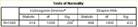Is it free to get my assignment evaluated?

Yes. No hidden fees. You pay for the solution only, and all the explanations about how to run it are included in the price. It takes up to 24 hours to get a quote from an expert. In some cases, we can help you faster if an expert is available, but you should always order in advance to avoid the risks. You can place a new order here.

How much does it cost?

The cost depends on many factors: how far away the deadline is, how hard/big the task is, if it is code only or a report, etc. We try to give rough estimates here, but it is just for orientation (in USD):

 Regular homework \$20 - \$150 Advanced homework \$100 - \$300 Group project or a report \$200 - \$500 Mid-term or final project \$200 - \$800 Live exam help \$100 - \$300 Full thesis \$1000 - \$3000

How do I pay?

Credit card or PayPal. You don't need to create/have a Payal account in order to pay by a credit card. Paypal offers you "buyer's protection" in case of any issues.

Why do I need to pay in advance?

We have no way to request money after we send you the solution. PayPal works as a middleman, which protects you in case of any disputes, so you should feel safe paying using PayPal.

Do you do essays?

No, unless it is a data analysis essay or report. This is because essays are very personal and it is easy to see when they are written by another person. This is not the case with math and programming.

Why there are no discounts?

It is because we don't want to lie - in such services no discount can be set in advance because we set the price knowing that there is a discount. For example, if we wanted to ask for \$100, we could tell that the price is \$200 and because you are special, we can do a 50% discount. It is the way all scam websites operate. We set honest prices instead, so there is no need for fake discounts.

Do you do live tutoring?

No, it is simply not how we operate. How often do you meet a great programmer who is also a great speaker? Rarely. It is why we encourage our experts to write down explanations instead of having a live call. It is often enough to get you started - analyzing and running the solutions is a big part of learning.

What happens if I am not satisfied with the solution?

Another expert will review the task, and if your claim is reasonable - we refund the payment and often block the freelancer from our platform. Because we are so harsh with our experts - the ones working with us are very trustworthy to deliver high-quality assignment solutions on time.

Customer Feedback

"Thanks for explanations after the assignment was already completed... Emily is such a nice tutor! "

Order #13073